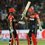# Looks Not So Big

$\large a = 1 + \dfrac1{2^2} + \dfrac1{3^2} + \cdots + \dfrac1{2016^2}$

Find the value of $\lfloor a \rfloor$.

Notation: $\lfloor \cdot \rfloor$ denotes the floor function.Note by Ayush G Rai
5 years ago

This discussion board is a place to discuss our Daily Challenges and the math and science related to those challenges. Explanations are more than just a solution — they should explain the steps and thinking strategies that you used to obtain the solution. Comments should further the discussion of math and science.

When posting on Brilliant:

• Use the emojis to react to an explanation, whether you're congratulating a job well done , or just really confused .
• Ask specific questions about the challenge or the steps in somebody's explanation. Well-posed questions can add a lot to the discussion, but posting "I don't understand!" doesn't help anyone.
• Try to contribute something new to the discussion, whether it is an extension, generalization or other idea related to the challenge.
• Stay on topic — we're all here to learn more about math and science, not to hear about your favorite get-rich-quick scheme or current world events.

MarkdownAppears as
*italics* or _italics_ italics
**bold** or __bold__ bold
- bulleted- list
• bulleted
• list
1. numbered2. list
1. numbered
2. list
Note: you must add a full line of space before and after lists for them to show up correctly
paragraph 1paragraph 2

paragraph 1

paragraph 2

[example link](https://brilliant.org)example link
> This is a quote
This is a quote
    # I indented these lines
# 4 spaces, and now they show
# up as a code block.

print "hello world"
# I indented these lines
# 4 spaces, and now they show
# up as a code block.

print "hello world"
MathAppears as
Remember to wrap math in $$ ... $$ or $ ... $ to ensure proper formatting.
2 \times 3 $2 \times 3$
2^{34} $2^{34}$
a_{i-1} $a_{i-1}$
\frac{2}{3} $\frac{2}{3}$
\sqrt{2} $\sqrt{2}$
\sum_{i=1}^3 $\sum_{i=1}^3$
\sin \theta $\sin \theta$
\boxed{123} $\boxed{123}$

## Comments

Sort by:

Top Newest

The answer is 1.

Note that if $s_n=\sum_{r=1}^n \frac{1}{r^2} \quad \forall n \in \mathbb{N}-\{1\} \\ s_1=1$ then $\{s_n\}_{n=1}^{\infty}$ is a strictly increasing sequence that which is always less than 2, that is, $n

Here are the proofs:

The first statement is true as $s_m$ contains more number of positive terms than $s_n$.

To prove the next assertion, note that \begin{aligned}0&

Now, $1=s_1

Note:

$s_{2016}<\lim_{n \to \infty} s_n = \frac{\pi^2}{6} < 1.645$

Refer here for the proof of this.

- 5 years ago

Log in to reply

Nice solution..+1.I guessed it anyway

- 5 years ago

Log in to reply

Try to answer other problems of the set.You are tooooo good in solving problems.

- 5 years ago

Log in to reply

Will try after jee advanced :)

- 5 years ago

Log in to reply

How much did you get in jee-main?

- 5 years ago

Log in to reply

Not so good... 250.

- 5 years ago

Log in to reply

That is pretty good. well i have a long way to go.I'm still in 9th std going to the 10th.

- 5 years ago

Log in to reply

I think the answer is 2.

- 5 years ago

Log in to reply

This is again for NMTC lvl 2 2016

- 5 years ago

Log in to reply

no it is of 2015

- 5 years ago

Log in to reply

Oh sorry I meant the previous year IE 2015....I know because I appeared...

- 5 years ago

Log in to reply

even I appeared

- 5 years ago

Log in to reply

How many could you solve??

- 5 years ago

Log in to reply

same as you...1 and a half

- 5 years ago

Log in to reply

how much problems could you solve??

- 5 years ago

Log in to reply

1 and a half....definitely not so good...One of my friends solved about 3 and qualified

- 5 years ago

Log in to reply

×

Problem Loading...

Note Loading...

Set Loading...